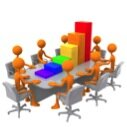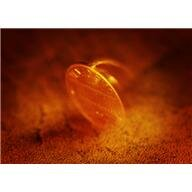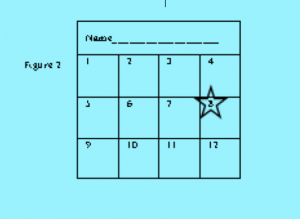# Category: Math Activities

## Enjoy Making Bar GraphsUsing graphs to represent data is an important feature of teaching math to elementary school students. Graphs come in many different shapes and sizes and can convey numerous types of information. As students progress through Math, they will encounter graphs in increasing complexity and will be asked to interpret data from graphs, draw conclusions from graphs, and even extrapolate information. Introducing graphing can be done through fun, interactive games that bring Math to life.  (photo: www.commons.wikimedia.org/wiki/File:3D_Bar_Graph_Meeting.jpg (10/27/13 7:15pm)

In this activity students will explore the how to collect and graph discrete data.

Materials:   Chart Paper, Markers

Instructions:

1. In the classroom, section off 4 distinct areas. Using corners of the classroom is the easiest way to do this.
2. Ask the students: “What is your favorite dessert? If you like cake, go to Corner #1; if you like cookies, go to Corner #2; if you like ice cream, go to Corner #3; if you like candy, go to Corner #4.
3. Allow time for students to decide which dessert they prefer and then record the number of students in each corner.
4. On the chart paper, have 4 columns, one for each dessert option. Write the number of students in the corresponding column.
5. Explain to the class that they just collected data on the type of dessert their classmates like. You may consider saying, “Data can be in the form of numbers or words, and in this case, we determined how many of you like each type of dessert. Next, we are going to do a graph, which is similar to a picture, showing the data we just collected.”
6. Create the axis of the graph, labeling the number of students on the vertical axis (y-axis) and the type of dessert on the horizontal axis (x-axis).
7. Mark the y-axis according to provide enough numbers to represent the numbers of students in each category. Draw in the bars to the corresponding number for each dessert type. For younger students, consider using stickers to represent the bars of the graph and have each student place a sticker in the dessert column they prefer.

Graphing is essential to building scientific knowledge and understanding as well as Math comprehension. For more interesting graphing activities, visit:

And for more of our Fun Learning Math Games, you can visit here:

www.math-lessons.ca/activities/index.html

www.math-lessons.ca/activities/FractionsBoard5.html

www.math-lessons.ca/activities/Geometry.html

http://www.literature-enrichment.com/

## Learning Measurement in Elementary MathAre you finished administering the same old paper and pencil tests to your students? Instead, why don’t you allow you students to demonstrate what they have learned? Here is a great way to assess your students’ learning with measurement units, using performance assessment.

We find that it is easiest to set the desks up in a circle so that students can move clockwise and there is no confusion as to where to move next. Use large index cards to number the problems and describe the task the student must complete while at that desk. A different problem is presented at each desk. Use a numbered index card to clearly indicate the problem number. (See Figure 1 for an example.)

Record The Value of The Five Coins

Students start at their own desk, recording the answer for that problem. All students will start on a different number. It may be helpful to provide young students a numbered table to record their answers. I find it is helpful to have students put a star on the number problem that is set up at their desk so they start recording answers in the correct box. (See figure 2 for an example).Here is an example of the types of problems that can be used in this assessment. The materials needed at each desk are indicated in the parentheses.

Desk 1 – (coins) Record the value of the five coins.

Desk 2 – (clock) Record the time shown on the clock.

Desk 3 – (pencil, ruler) Measure the length of a pencil to the nearest half-inch.

Desk 4 – (calendar) Record the date of the second Wednesday in March.

Desk 5 – (apple, scale, gram weights) Find the weight of the apple to the nearest gram.

Desk 6 – (clock) Identify the time on the clock. What time was it 15 minutes earlier?

Desk 7 – (calendar) Today’s date is highlighted on the calendar. Marie’s birthday is in 12 days. What is the date of Marie’s birthday?

Desk 8 – (blackboard eraser, ruler) Measure the length of the eraser to the nearest centimeter.

Desk 9 – (clock) Charles started his homework at 3:45. He worked for 20 minutes. What time was it when he finished his homework?

Desk 10 – (1-inch squares and an outline of a large rectangle) Lay the squares on the rectangle to determine the area of the rectangle. Remember to label your answer with square inches.

For more fun and interesting Learning Math Games, you can visit us here:
http://www.math-lessons.ca/activities/FractionsBoard5.html
http://www.math-lessons.ca/timestables/times-tables.html
http://www.math-lessons.ca/activities/FractionsBoard4.html
http://www.math-lessons.ca/index.html